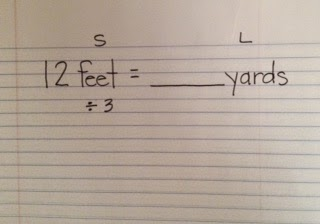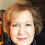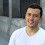[Boxedwidth]

No

## Grid Slider Styles

[style1][caption2]

No

## Display Instagram Footer

By 5th grade, students have been making measurement conversions for a while.  I still like to review the history of both measurement systems, both standard and metric, the three measurement attributes we use (standard: length, capacity, weight, and metric: length, volume, and mass), and the basic facts.  I supply students with this information in their math notebooks.

When making conversions, I like to show a number of examples and let kids tell me what's happening when we convert from one unit to another.  For example, I might put this on the SmartBoard:
12 feet = 4 yards
60 inches = 5 feet
8 quarts = 2 gallons
4,000 pounds = 2 tons
1.  What can you tell me about the change in units: did we convert from large to small or small to large?  In this example, we converted smaller units to larger units.

2.  Then, I ask what happened to the numbers.  They reply that the numbers got smaller.  Through discussion, I want students to see that when you take a lot of little things and put them in larger groups, you'll end up with fewer groups.  For example, taking 12 feet (a smaller unit) and grouping them into yards (a larger unit), I'll end up with fewer yards than feet.

3.   The last question, then, is: What operation did I use to do that?  The answer is divide.  When we divide, we'll end up with a quotient that's smaller than our dividend.

Of course, it's just the opposite when we convert from larger to smaller.
6 yards = 18 feet
4 feet = 48 inches
6 gallons = 24 quarts
3 tons = 6,000 pounds
1.  We converted from larger to smaller.
2.  The numbers got bigger.
3.  We multiplied to make that happen.

Still, it can take some time for students to process this concept so that it's automatic for them.  In the meantime, we still have to make conversions.  Several years ago, I learned this memory trick and it's really been helpful.  A picture is worth a thousand words:
We focus on the beginning letters of the phrases:
Snakes Love Ditches = When we convert from small units to large units, we divide, and
Large Snakes Multiply = When we convert from large units to small units, we multiply.
To pull it all together, the display looks like this:
I don't remember when we decided to name the snakes "Monty", but there it is!
A student's work looks like this:Write the problem and label the units as small or large.Say the correct "ditty": Snakes love ditches" and record the operation you'll use.State the basic fact: There are 3 feet in one yard.  Record it.Use the number you've been given; in this case, 12 feet.  Almost done!This is a long blog post, but when it comes to the conversions, it honestly can be done in 15 seconds.  Give it a try and let me know how it works for you. Complete the math.  You've made a successful conversion!Share :

1.This is great - can't wait to use with my class. Thank you so much for sharing.

2.That's wonderful! I love this strategy - best of luck to you and your students!

3.You could actually convert centimeter to inches by just using simple calculations. Conversions don't include any difficult steps of divisions or multiplications. You may either calculate it manually with pen and paper or use a calculator . cm to inches

4.This comment has been removed by a blog administrator.